Courses

# Test: Fluid Mechanics (Level -II)- 2

## 25 Questions MCQ Test Mechanical Engineering SSC JE (Technical) | Test: Fluid Mechanics (Level -II)- 2

Description
This mock test of Test: Fluid Mechanics (Level -II)- 2 for Mechanical Engineering helps you for every Mechanical Engineering entrance exam. This contains 25 Multiple Choice Questions for Mechanical Engineering Test: Fluid Mechanics (Level -II)- 2 (mcq) to study with solutions a complete question bank. The solved questions answers in this Test: Fluid Mechanics (Level -II)- 2 quiz give you a good mix of easy questions and tough questions. Mechanical Engineering students definitely take this Test: Fluid Mechanics (Level -II)- 2 exercise for a better result in the exam. You can find other Test: Fluid Mechanics (Level -II)- 2 extra questions, long questions & short questions for Mechanical Engineering on EduRev as well by searching above.
QUESTION: 1

Solution:
QUESTION: 2

Solution:
QUESTION: 3

### A large metacentric height in a vessel

Solution:
QUESTION: 4

The time of oscillation of a floating body is given by Where
K = Radius of Gyration of the floating body about its centre of gravity
h = Metacentric height of the floating body

Solution:
QUESTION: 5

A cylindrical body of cross-sectional area A height H and densityrs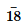is immersed to a depth h in a liquid of density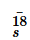r and tied down to bottom with a string then the tension in the string is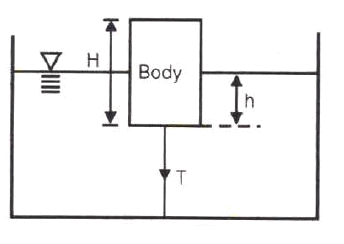Solution:
QUESTION: 6

There is no geometrical distinction between the streamline, pathline and streak line in case of

Solution:
QUESTION: 7

Stream line, path line and streak line are identical when the

Solution:
QUESTION: 8

Match List I with List II and select the correct answer using the codes given below the lists: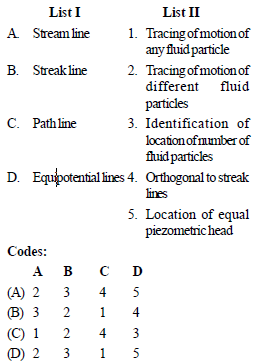Solution:
QUESTION: 9

The continuity equation in differential form is

Solution:
QUESTION: 10

Under which of the following conditions will the equation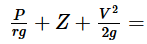constant, will be valid in the whole flow field ?

1. Flow is rotational
2. Flow is irrotational
3. Flow is incompressible
5. Flow is laminar

Solution:
QUESTION: 11

Venturimeter consists of following parts :
1. Diverging part
2. Throat
3. Main inlet pipe
4. Short converging part
Select the correct sequence of parts

Solution:
QUESTION: 12

The range for coefficient of discharge (Cd) for a venturimeter is

Solution:
QUESTION: 13

In the siphon in figure below assuming ideal flow, the pressure PB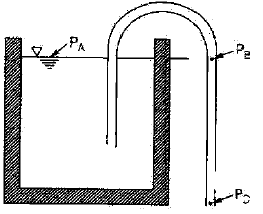Solution:
QUESTION: 14

The flow of fluid through a pipe is laminar when

Solution:
QUESTION: 15

An oil of kinematic viscosity 0.5 stokes flows through a pipe of 4 cm diameter. The flow is critical at a velocity of about

Solution:
QUESTION: 16

In the Navier-Stokes equations, the forces considered are

Solution:
QUESTION: 17

Navier-Stokes equation represents the conservation of

Solution:

The Navier-Stokes equations govern the motion of fluids and can be seen as Newton's second law of motion for fluids. ... The Navier-Stokes equations represent the conservation of momentum, while the continuity equation represents the conservation of mass.

QUESTION: 18

For laminar flow between two fixed parallel plates, the flow velocity

Solution:
QUESTION: 19

Capillary tube viscometers used for measurement of viscosity are based on

Solution:
QUESTION: 20

An incompressible fluid (kinematic viscosity, 7.4 × 10–7 m2/s, specific gravity, 8.88) is held between two parallel plates. If the top plate is moved with a velocity of 0.5 m/s while the bottom one is held stationary, the fluid attains a linear velocity profile in the gap of 0.5 mm between these plates; the shear stress in Pascals on the surface of top plate is

Solution:
QUESTION: 21

Pressure drop in a 100 mm diameter horizontal pipe is 50 kPa over a length of 10 m. The shear stress at the pipe wall is

Solution:
QUESTION: 22

The pressure drop for a relatively low Reynolds number flow in a 600 mm, 30 m long pipe line is 70 kPa. What is the wall shear stress

Solution:
QUESTION: 23

When the hydrodynamic boundary layer thickness (δ), which is the distance from the surface at which the local velocity (u) reaches 99% of the free-stream velocity, then

Solution:
QUESTION: 24

Shear velocity is

Solution:
QUESTION: 25

The head loss in a sudden expansion from 5 cm diameter to 10 cm diameter pipe, in terms of velocity V1 in the 5 cm pipe

Solution: